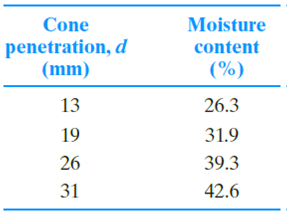Chapter 4, Problem 4.7PPrinciples of Geotechnical Enginee...

9th Edition
Braja M. Das + 1 other
ISBN: 9781305970939

Solutions

Chapter
SectionPrinciples of Geotechnical Enginee...

9th Edition
Braja M. Das + 1 other
ISBN: 9781305970939
Textbook Problem

Following results are obtained for a liquid limit test using a fall cone device. Estimate the liquid limit of the soil and the flow index.To determine

Find the liquid limit and flow index of the soil.

Explanation

Draw the graph between the cone penetration and the moisture content as in Figure 1.

Refer Figure 1.

The liquid limit obtained from the fall cone test is 33.8 %.

Therefore, the liquid limit of the soil (LL) is 33.8%_.

Determine the flow index for the soil (IF) using the relation.

IFC=w2(%)w1(%)logd2logd1

Here, w2 is the moisture content of soil at cone penetration of d2 and w2 is the moisture content of soil at cone penetration of d2

Still sussing out bartleby?

Check out a sample textbook solution.

See a sample solution

The Solution to Your Study Problems

Bartleby provides explanations to thousands of textbook problems written by our experts, many with advanced degrees!

Get Started

Solve Problem 20.22 using Excel.

Engineering Fundamentals: An Introduction to Engineering (MindTap Course List)

Determine the resultant of the four forces.

International Edition---engineering Mechanics: Statics, 4th Edition

Describe the strategy of acceptance.

Management Of Information Security

What e three types of sampling? Which one would you use to analyze data input errors?

Systems Analysis and Design (Shelly Cashman Series) (MindTap Course List)

What is the purpose of grooving a joint before welding on thick plate?

Welding: Principles and Applications (MindTap Course List)

Identify ways to avoid malware when using a mobile device.

Enhanced Discovering Computers 2017 (Shelly Cashman Series) (MindTap Course List)